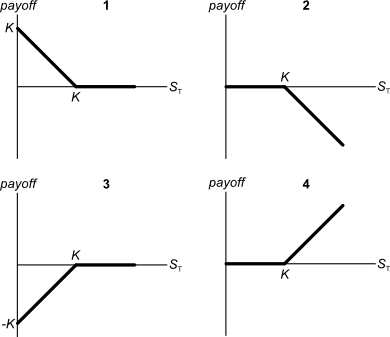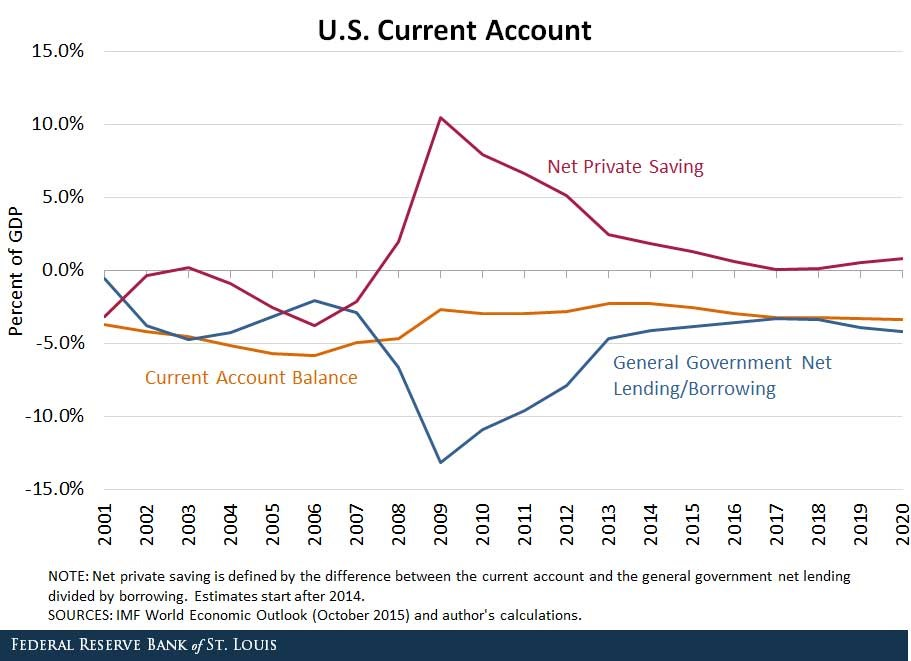# Fight Finance

#### CoursesTagsRandomAllRecentScores

The following is the Dividend Discount Model (DDM) used to price stocks:

$$P_0 = \frac{d_1}{r-g}$$

Assume that the assumptions of the DDM hold and that the time period is measured in years.

Which of the following is equal to the expected dividend in 3 years, $d_3$?

Below are 4 option graphs. Note that the y-axis is payoff at maturity (T). What options do they depict? List them in the order that they are numbered.For certain shares, the forward-looking Price-Earnings Ratio ($P_0/EPS_1$) is equal to the inverse of the share's total expected return ($1/r_\text{total}$).

For what shares is this true?

Assume:

• The general accounting definition of 'payout ratio' which is dividends per share (DPS) divided by earnings per share (EPS).
• All cash flows, earnings and rates are real.

If the AUD appreciates against the USD, the American terms quote of the AUD will or ?

One of the reasons why firms may not begin projects with relatively small positive net present values (NPV's) is because they wish to maximise the value of their:

One year ago you bought $100,000 of shares partly funded using a margin loan. The margin loan size was$70,000 and the other $30,000 was your own wealth or 'equity' in the share assets. The interest rate on the margin loan was 7.84% pa. Over the year, the shares produced a dividend yield of 4% pa and a capital gain of 5% pa. What was the total return on your wealth? Ignore taxes, assume that all cash flows (interest payments and dividends) were paid and received at the end of the year, and all rates above are effective annual rates. Hint: Remember that wealth in this context is your equity (E) in the house asset (V = D+E) which is funded by the loan (D) and your deposit or equity (E). A$100 stock has a continuously compounded expected total return of 10% pa. Its dividend yield is 2% pa with continuous compounding. What do you expect its price to be in 2.5 years?

A one year European-style put option has a strike price of $4. The option's underlying stock pays no dividends and currently trades at$5. The risk-free interest rate is 10% pa continuously compounded. Use a single step binomial tree to calculate the option price, assuming that the price could rise to $8 $(u = 1.6)$ or fall to$3.125 $(d = 1/1.6)$ in one year. The put option price now is:

You're thinking of buying an investment property that costs $1,000,000. The property's rent revenue over the next year is expected to be$50,000 pa and rent expenses are $20,000 pa, so net rent cash flow is$30,000. Assume that net rent is paid annually in arrears, so this next expected net rent cash flow of $30,000 is paid one year from now. The year after, net rent is expected to fall by 2% pa. So net rent at year 2 is expected to be$29,400 (=30,000*(1-0.02)^1).

The year after that, net rent is expected to rise by 1% pa. So net rent at year 3 is expected to be $29,694 (=30,000*(1-0.02)^1*(1+0.01)^1). From year 3 onwards, net rent is expected to rise at 2.5% pa forever. So net rent at year 4 is expected to be$30,436.35 (=30,000*(1-0.02)^1*(1+0.01)^1*(1+0.025)^1).

Assume that the total required return on your investment property is 6% pa. Ignore taxes. All returns are given as effective annual rates.

What is the net present value (NPV) of buying the investment property?

Observe the below graph of the US current account surplus as a proportion of GDP.Define lending as buying (or saving or investing in) debt and equity assets.

The sum of US ‘net private saving’ plus ‘net general government lending’ equals the US: